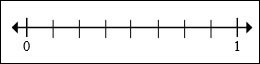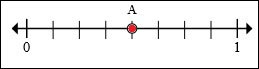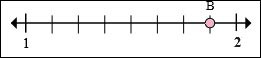# Fractional Position on a Number Line

#### Complete Python Prime Pack

9 Courses     2 eBooks

#### Artificial Intelligence & Machine Learning Prime Pack

6 Courses     1 eBooks

#### Java Prime Pack

9 Courses     2 eBooks

We show whole numbers on the number line. The number line between successive whole numbers is divided into as many equal parts or units as required using marks. Each unit thus can show fractional numbers on the number line.

In the number line below, there are 8 parts between 0 and 1. So each of the part is a 1/8th and the divisions are 1/8th unit apart.What is the position of A on the number line below?### Solution

Step 1:

We must count the number of parts between 0 and 1.

The marks divide one unit into 8 parts and thus each of this are 1/8th and are 1/8th unit apart.

Step 2:

We see that A is on the 4th mark to the right of 1. So A is at 4/8 mark on the number line

What is the position of B on the number line below?

Write your answer as a fraction or mixed number.### Solution

Step 1:

We must count the number of parts between 1 and 2.

So the marks divide one unit into 8 parts and thus each of this are 1/8th and are 1/8th unit apart.

Step 2:

We see that B is on the 7th mark to the right of 1. So B is at 1$\frac{7}{8}$ on the number line.